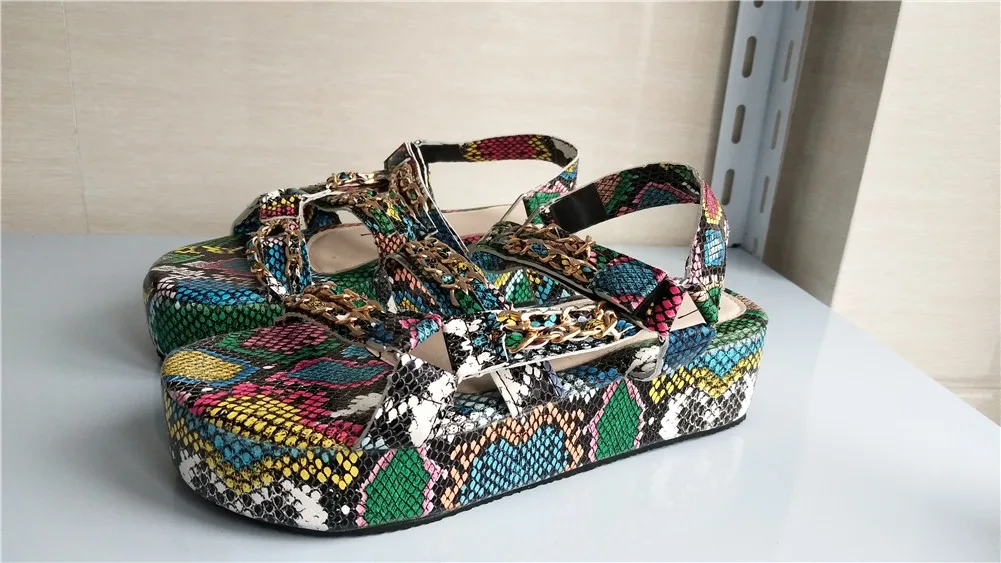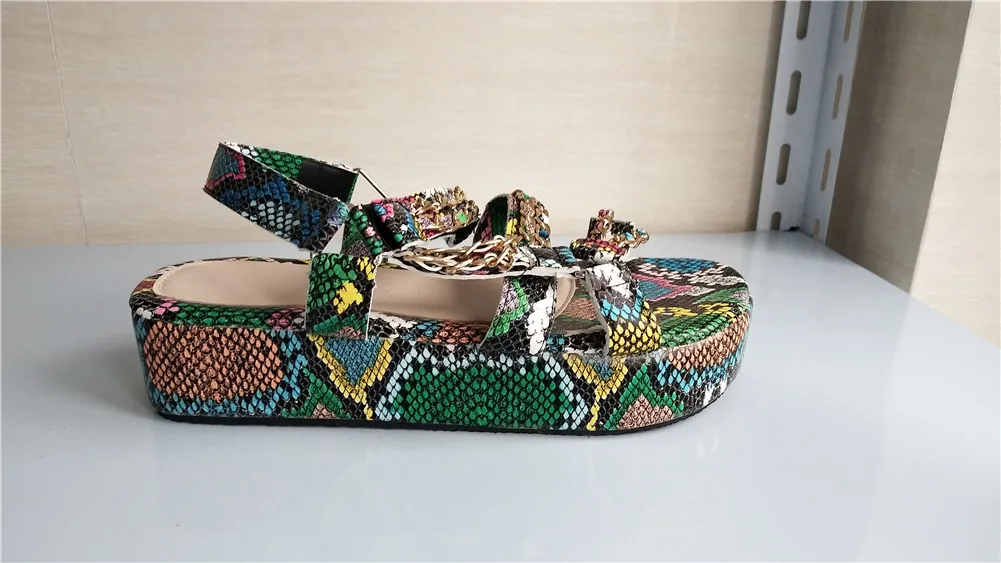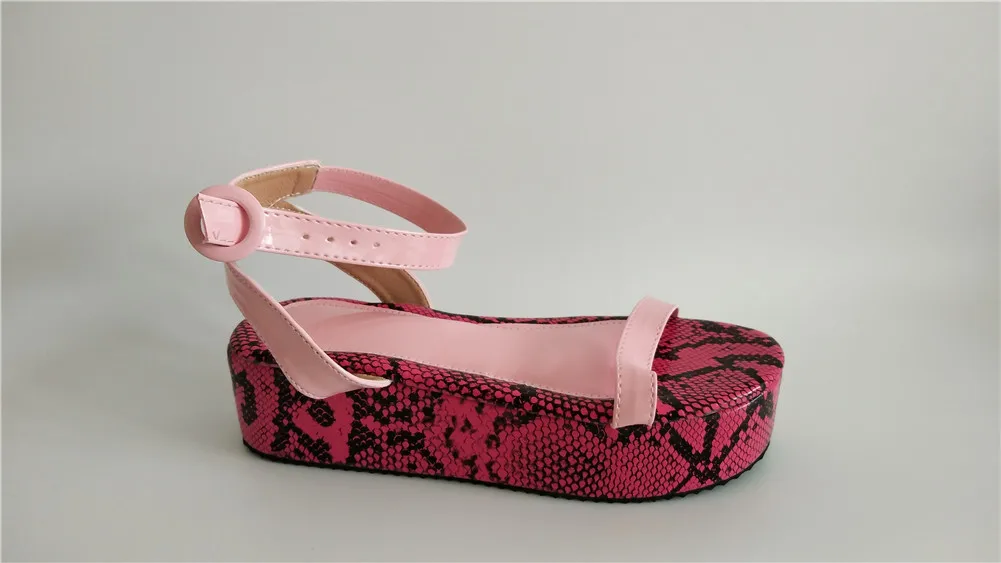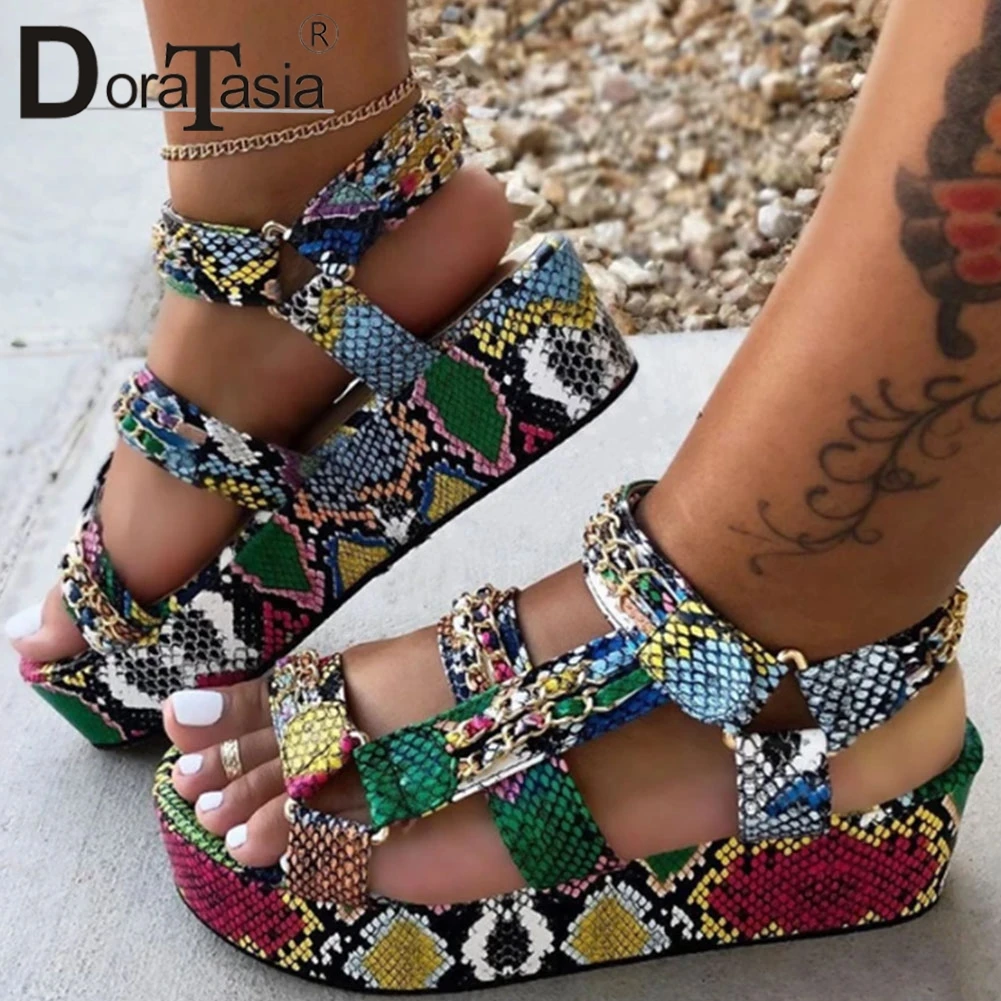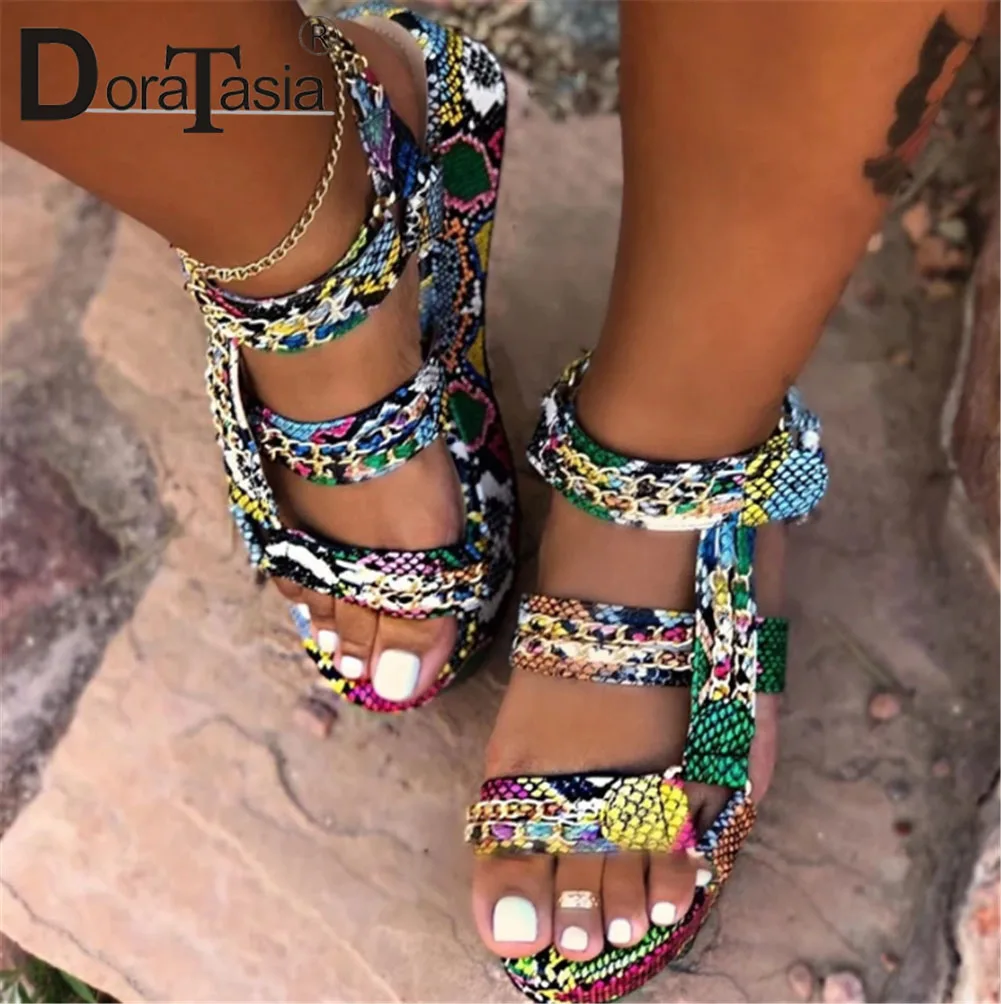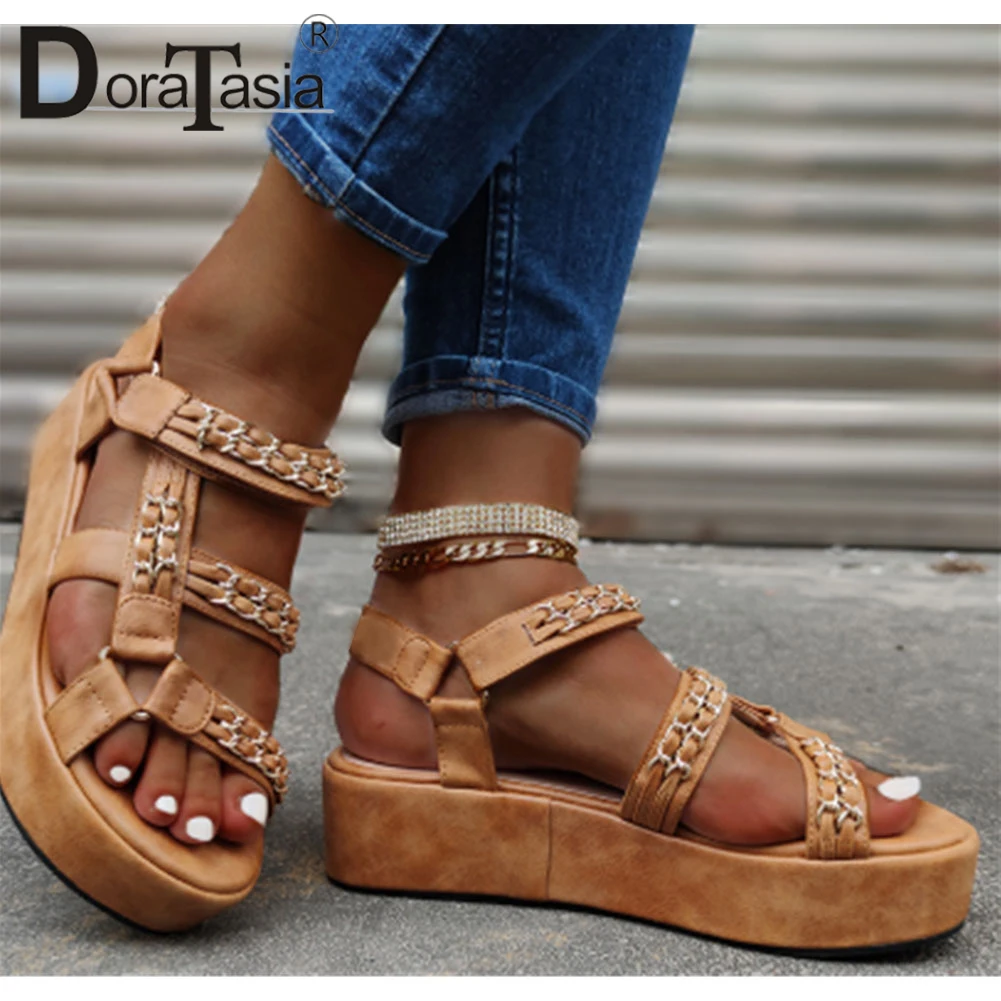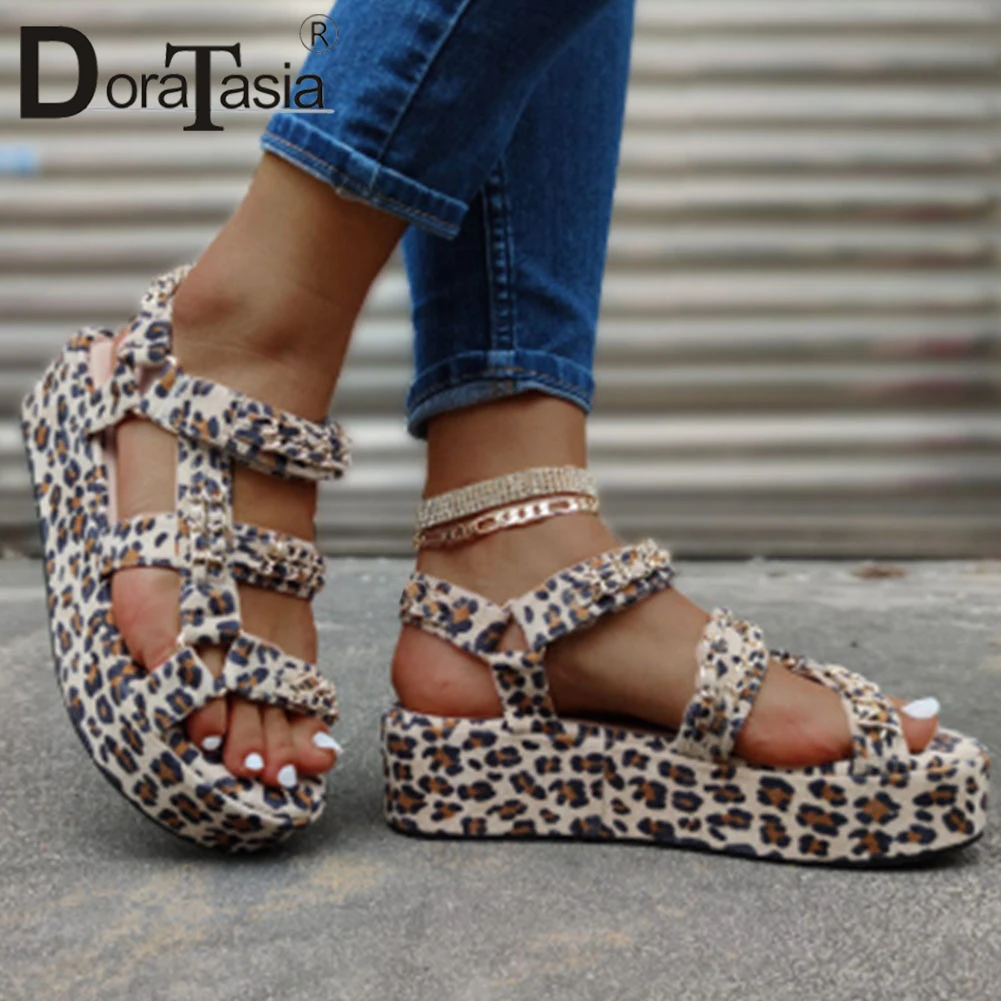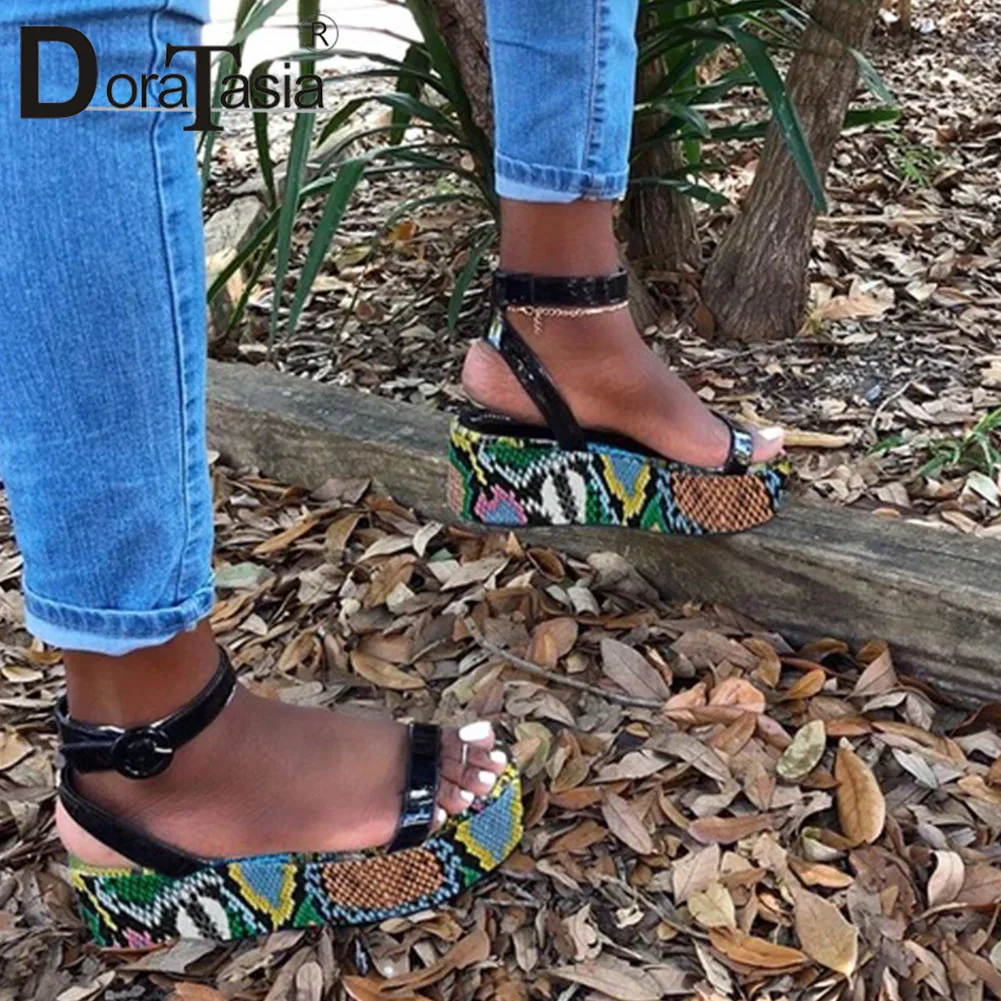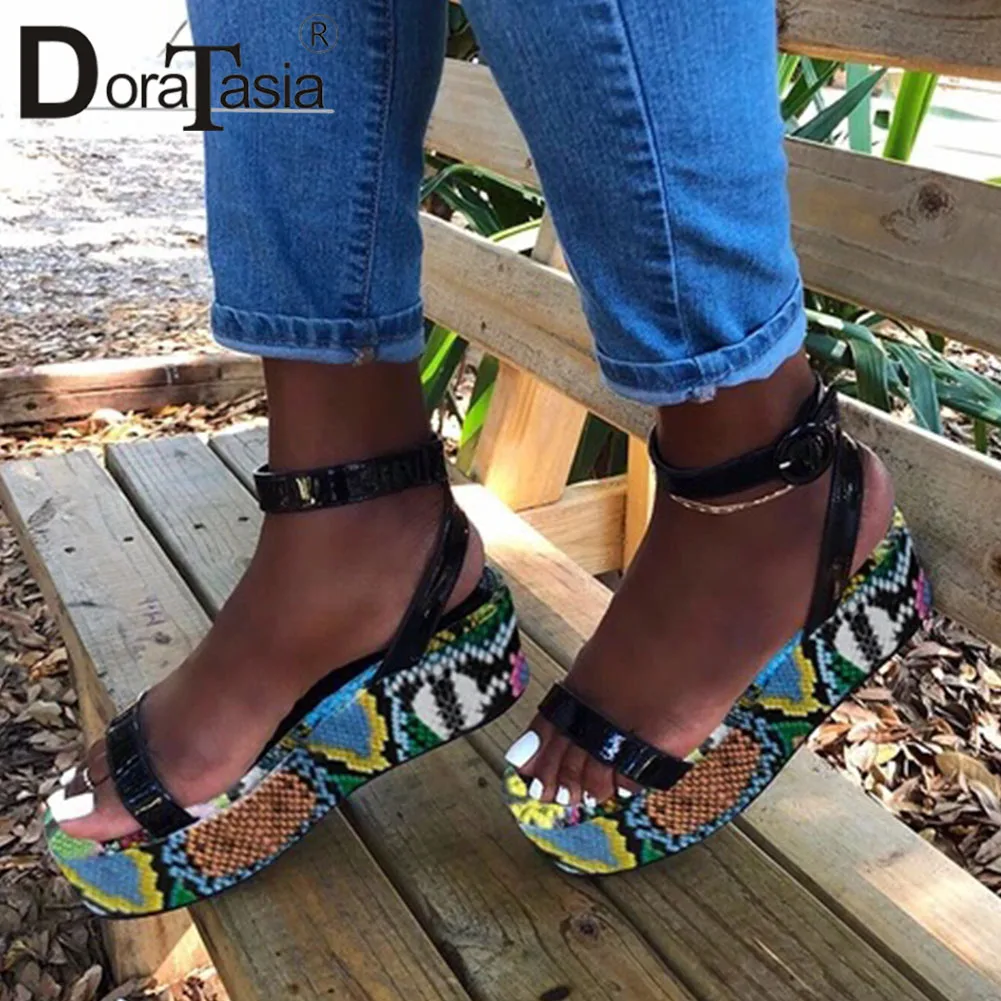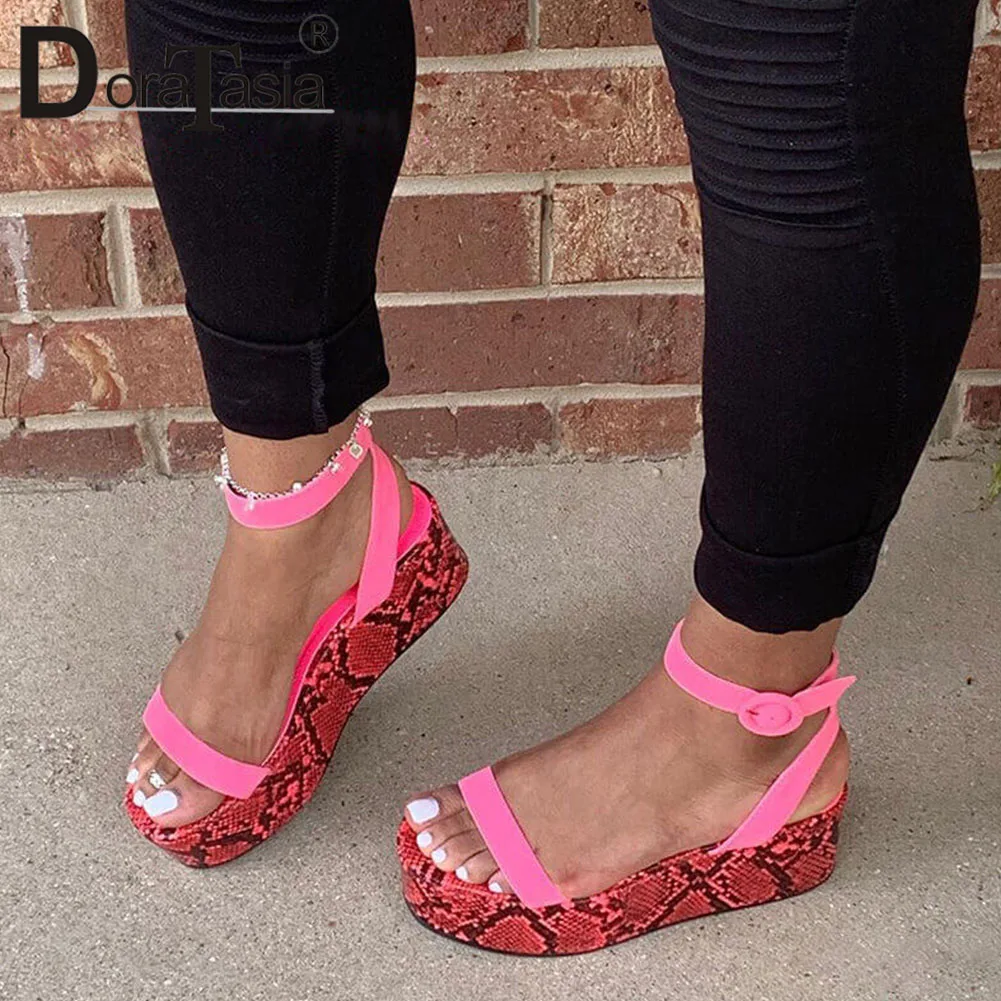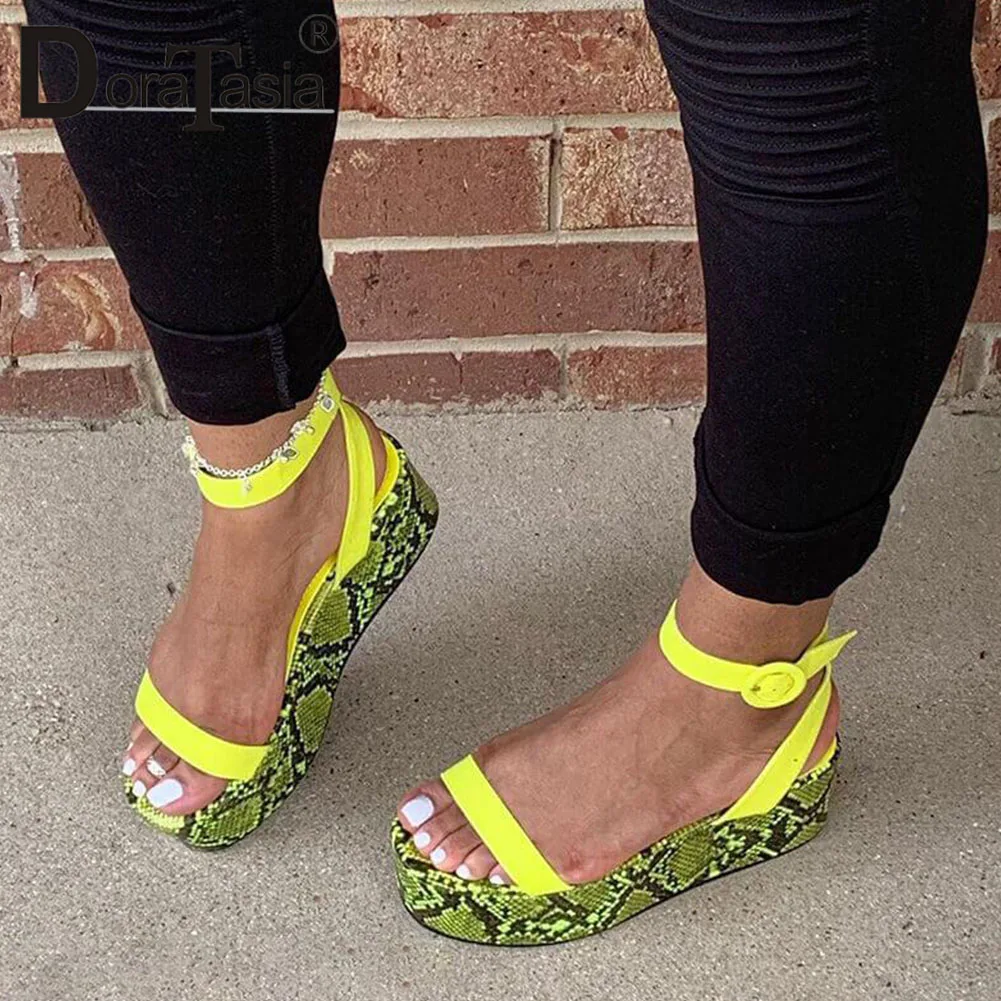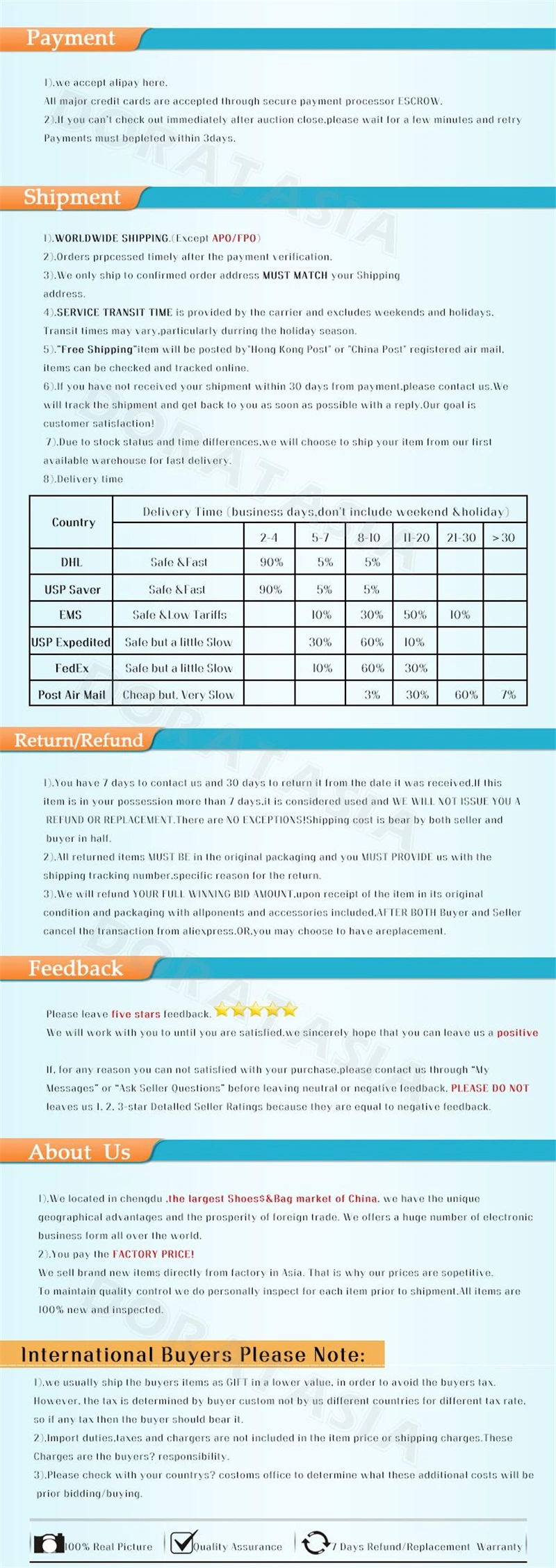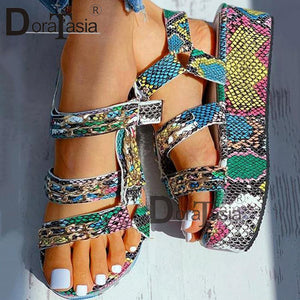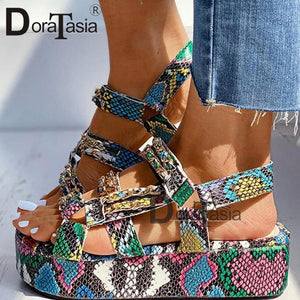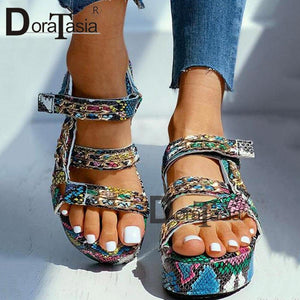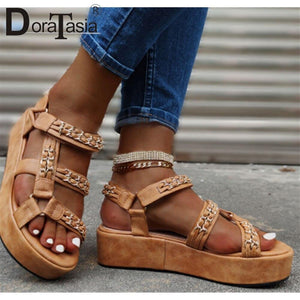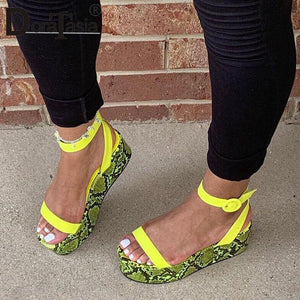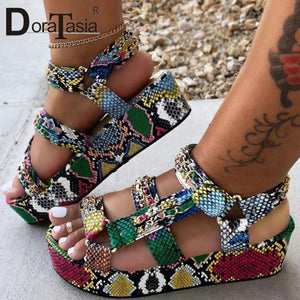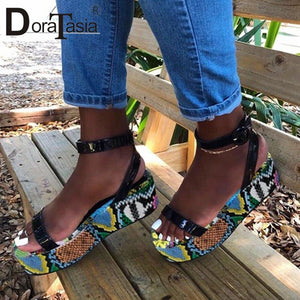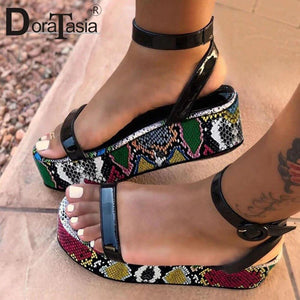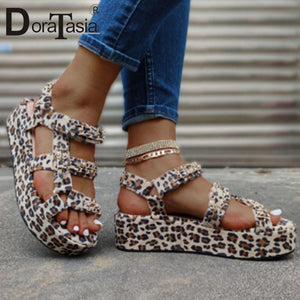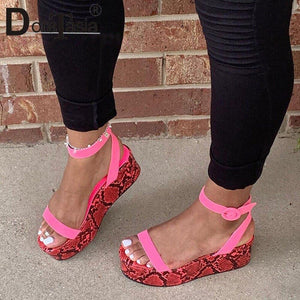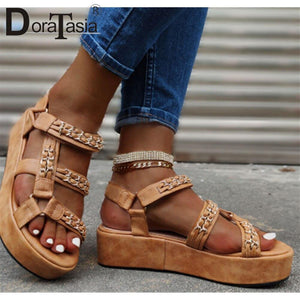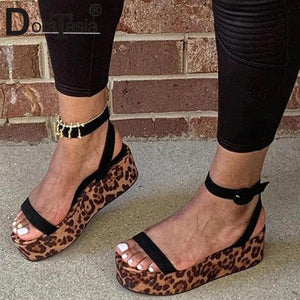Regular price £37.84
Unit price  per
Shipping calculated at checkout.

1. Our product size standard:
Size 2 =CN32=210mm (bare feet length)
Size 3 =CN33=215mm (bare feet length)
Size 4 =CN34=220mm (bare feet length)
Size 5 =CN35=225mm (bare feet length)
Size 6 =CN36=230mm (bare feet length)
Size 6.5=CN37=235mm (bare feet length)
Size 7.5=CN38=240mm (bare feet length)
Size 8.5=CN39=245mm (bare feet length)
Size 9 =CN40=250mm (bare feet length)
Size 9.5=CN41=255mm (bare feet length)
Size 10 =CN42=260mm (bare feet length)
Size 10.5=CN43=265mm (bare feet length)

Size 11=CN44=270mm (bare feet length)

2.notice:because of special material,the pattern is random,the main color might be different sometimes.hope you can know it ,thank you!

the real pictures